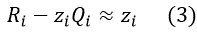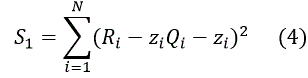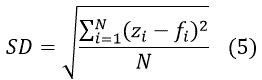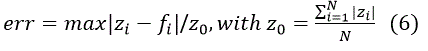General information Description Let us have N data points with coordinates xi, yi, zi.The main purpose of this calculation is to obtain a relatively simple formula of data fitting z=f(x,y). We apply the generalized Pade functions for the approximation of data in the following form: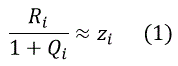where R(x, y), Q(x,y) are polynomial functions, Ri and Qi are their values calculated at i-th point. Pade functions have the following advantages: They have the same or better convergence compared to the power series. They can include functions with singularities and asymptotic behaviour of a function
 You can find an excellent summary of properties of Pade functions here.
 Methodology The residual sum of squares (RSS) corresponding to (1) is the following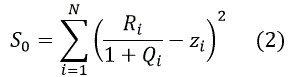Minimization of S0 leads to a system of nonlinear equations with respect to coefficients of polynomial functions, therefore the equation (1) is presented in the equivalent form